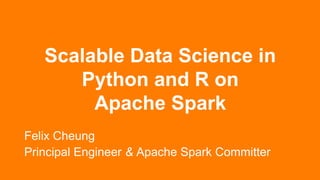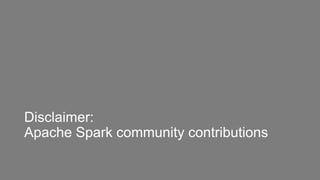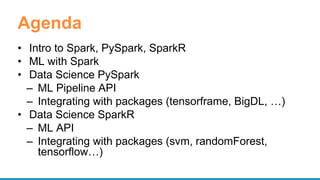Successfully reported this slideshow.

# Scalable Data Science in Python and R on Apache Spark×

1 of 68
1 of 68

# Scalable Data Science in Python and R on Apache Spark

In the world of Data Science, Python and R are very popular. Apache Spark is a highly scalable data platform. How could a Data Scientist integrate Spark into their existing Data Science toolset? How does Python work with Spark? How could one leverage the rich 10000+ packages on CRAN for R?

We will start with PySpark, beginning with a quick walkthrough of data preparation practices and an introduction to Spark MLLib Pipeline Model. We will also discuss how to integrate native Python packages with Spark.

Compare to PySpark, SparkR is a new language binding for Apache Spark and it is designed to be familiar to native R users. In this talk we will walkthrough many examples how several new features in Apache Spark 2.x will enable scalable machine learning on Big Data. In addition to talking about the R interface to the ML Pipeline model, we will explore how SparkR support running user code on large scale data in a distributed manner, and give examples on how that could be used to work with your favorite R packages.

Python
R
Apache Spark
ML
DL

In the world of Data Science, Python and R are very popular. Apache Spark is a highly scalable data platform. How could a Data Scientist integrate Spark into their existing Data Science toolset? How does Python work with Spark? How could one leverage the rich 10000+ packages on CRAN for R?

We will start with PySpark, beginning with a quick walkthrough of data preparation practices and an introduction to Spark MLLib Pipeline Model. We will also discuss how to integrate native Python packages with Spark.

Compare to PySpark, SparkR is a new language binding for Apache Spark and it is designed to be familiar to native R users. In this talk we will walkthrough many examples how several new features in Apache Spark 2.x will enable scalable machine learning on Big Data. In addition to talking about the R interface to the ML Pipeline model, we will explore how SparkR support running user code on large scale data in a distributed manner, and give examples on how that could be used to work with your favorite R packages.

Python
R
Apache Spark
ML
DL

## More Related Content

### Scalable Data Science in Python and R on Apache Spark

1. 1. Scalable Data Science in Python and R on Apache Spark Felix Cheung Principal Engineer & Apache Spark Committer
2. 2. Disclaimer: Apache Spark community contributions
3. 3. Agenda • Intro to Spark, PySpark, SparkR • ML with Spark • Data Science PySpark – ML Pipeline API – Integrating with packages (tensorframe, BigDL, …) • Data Science SparkR – ML API – Integrating with packages (svm, randomForest, tensorflow…)
4. 4. What is Spark? • General-purpose cluster computing system
5. 5. Distributed Computation
6. 6. What are the ways to use Spark? • Ingestion – Batch – Streaming (low latency, static data) • ETL – SQL – Leveraging Catalyst Optimizer, Tungsten, CBO (cost-based optimizer in Spark 2.2/2.3) • Distributed ML
7. 7. Language Bindings
8. 8. PySpark • Python API with Pandas-like DataFrame • Interface to Pandas, numpy • cloudpickle to serialize functions/closures
9. 9. Architecture • Python classes • Py4J • Daemon process
10. 10. SparkR • R language APIs for Spark • Exposes Spark functionality in a R dplyr-like APIs • Runs as its own REPL sparkR • or as a R package loaded in IDEs like RStudio library(SparkR) sparkR.session()
11. 11. Architecture • Native R classes and methods • RBackend • Scala wrapper/helper (eg. ML Pipeline) www.slideshare.net/SparkSummit/07-venkataraman-sun
12. 12. High Performance • JVM processing, full access to DAG capabilities and Catalyst optimizer, predicate pushdown, code generation, etc. databricks.com/blog/2015/06/09/announcing-sparkr-r-on-spark.html
13. 13. ML/DL on Spark
14. 14. End-to-End Data-Machine Learning Pipeline • Ingestion • Data preprocessing, cleaning, ETL • Machine Learning – models, predictions • Serving
15. 15. Why in Spark? ETL Ingest Machine Learning Analytic
16. 16. Why in Spark • Existing workloads (eg. ETL) • Single application – Single language • Sampling, aggregation • Scale
17. 17. Spark in ML Architecture 1 ETL Ingest Machine Learning Serve
18. 18. Spark in ML Architecture 2 ETL Ingest Model Packages Packages Packages Packages Machine Learning Evaluate
19. 19. Spark in ML Architecture 3 ETL Ingest Model Machine Learning Serve
20. 20. PySpark for Data Science
21. 21. Spark ML Pipeline • Inspired by scikit-learn • Pre-processing, feature extraction, model fitting, validation stages • Transformer • Estimator • Evaluator • Cross-validation/hyperparameter tuning
22. 22. DataFrame Spark ML Pipeline Transformer EstimatorTransformer Feature engineering Modeling
23. 23. Classification: Logistic regression Binomial logistic regression Multinomial logistic regression Decision tree classifier Random forest classifier Gradient-boosted tree classifier Multilayer perceptron classifier One-vs-Rest classifier (a.k.a. One-vs-All) Naive Bayes Models Clustering: K-means Latent Dirichlet allocation (LDA) Bisecting k-means Gaussian Mixture Model (GMM) Collaborative Filtering: Alternating Least Squares (ALS) Regression: Linear regression Generalized linear regression Decision tree regression Random forest regression Gradient-boosted tree regression Survival regression Isotonic regression
24. 24. PySpark ML Pipline Model tokenizer = Tokenizer(inputCol="text", outputCol="words") hashingTF = HashingTF(inputCol=tokenizer.getOutputCol(), outputCol="features") lr = LogisticRegression(maxIter=10, regParam=0.001) pipeline = Pipeline(stages=[tokenizer, hashingTF, lr]) # Fit the pipeline to training documents. model = pipeline.fit(training) prediction = model.transform(test)
25. 25. Large Scale, Distributed • 2012 paper “Large Scale Distributed Deep Networks” Jeff Dean et al • Model parallelism • Data parallelism https://static.googleusercontent.com/media/research.google.com/en//archive/large_deep_networks_nips2012.pdf
26. 26. Spark as a Scheduler • Run external, often native packages • Data-parallel tasks • Distributed execution external data
27. 27. UDF • Register function to use in SQL – spark.udf.register("stringLengthInt", lambda x: len(x), IntegerType()) spark.sql("SELECT stringLengthInt('test')") • Function on RDD – rdd.map(lambda l: str(l.e)[:7]) – rdd.mapPartitions(lambda x: [(1,), (2,), (3,)])
28. 28. PySpark UDF Python Executor UDF Row Batch Row
29. 29. Considerations • Data transfer overhead (serialization/deserialization, network) • Native language (boxing, interpreted) • Lambda – one row at a time
30. 30. PySpark Vectorized UDF • Apache Arrow – in-memory columnar – Row batch – Zero copy – Future: IPC • SPARK-21190
31. 31. spark-sklearn • Train and evaluate multiple scikit-learn models – Single node parallel -> distributed • Dataframes -> numpy ndarrays or sparse matrices • GridSearchCV, gapply
32. 32. CaffeOnSpark • GPU • RDMA to sync DL models • MPI Allreduce http://yahoohadoop.tumblr.com/post/129872361846/large-scale-distributed-deep-learning-on-hadoop
33. 33. CaffeOnSpark def get_predictions(sqlContext, images, model, imagenet, lstmnet, vocab): rdd = images.mapPartitions(lambda im: predict_caption(im, model, imagenet, lstmnet, vocab)) ... return sqlContext.createDataFrame(rdd, schema).select("result.id", "result.prediction") def predict_caption(list_of_images, model, imagenet, lstmnet, vocab): out_iterator = [] ce = CaptionExperiment(str(model),str(imagenet),str(lstmnet),str(vocab)) for image in list_of_images: out_iterator.append(ce.getCaption(image)) return iter(out_iterator) https://github.com/yahoo/CaffeOnSpark/blob/master/caffe-grid/src/main/python/examples/ImageCaption.py
34. 34. BigDL https://www.slideshare.net/SparkSummit/bigdl-a-distributed-deep-learning-library-on-spark-spark-summit-east-talk-by-yiheng-wang
35. 35. BigDL • Distribute model training – P2P All Reduce – block manager as parameter server • Integration with Intel's Math Kernel Library (MKL) • Model Snapshot • Load Caffe/Torch Model
36. 36. BigDL https://www.slideshare.net/SparkSummit/bigdl-a-distributed-deep-learning-library-on-spark-spark-summit-east-talk-by-yiheng-wang
37. 37. BigDL - CNN MNIST def build_model(class_num): model = Sequential() # Create a LeNet model model.add(Reshape([1, 28, 28])) model.add(SpatialConvolution(1, 6, 5, 5).set_name('conv1')) model.add(Tanh()) model.add(SpatialMaxPooling(2, 2, 2, 2).set_name('pool1')) model.add(Tanh()) model.add(SpatialConvolution(6, 12, 5, 5).set_name('conv2')) model.add(SpatialMaxPooling(2, 2, 2, 2).set_name('pool2')) model.add(Reshape([12 * 4 * 4])) model.add(Linear(12 * 4 * 4, 100).set_name('fc1')) model.add(Tanh()) model.add(Linear(100, class_num).set_name('score')) model.add(LogSoftMax()) return model https://github.com/intel-analytics/BigDL-Tutorials/blob/master/notebooks/neural_networks/cnn.ipynb lenet_model = build_model(10) state = {"learningRate": 0.4, "learningRateDecay": 0.0002} optimizer = Optimizer( model=lenet_model, training_rdd=train_data, criterion=ClassNLLCriterion(), optim_method="SGD", state=state, end_trigger=MaxEpoch(20), batch_size=2048) trained_model = optimizer.optimize() predictions = trained_model.predict(test_data)
38. 38. mmlspark • Spark ML Pipeline model + rich data types: images, text • Deep Learning with CNTK – Train on GPU edge node • Transfer Learning # Initialize CNTKModel and define input and output columns cntkModel = CNTKModel().setInputCol("images").setOutputCol("output").setModelLocation(model File) # Train on dataset with internal spark pipeline scoredImages = cntkModel.transform(imagesWithLabels)
39. 39. tensorframes • DataFrame -> TensorFlow • Hyperparameter tuning • Apply trained model at scale • Row or block operations • JVM to C++ (bypassing Python)
40. 40. tensorframes
41. 41. spark-deep-learning • Spark ML Pipeline model + complex data: image – Transformer • DL featurization • Transfer Learning • Model as SQL UDF • Later : hyperparameter tuning
42. 42. spark-deep-learning predictor = DeepImagePredictor(inputCol="image", outputCol="predicted_labels", modelName="InceptionV3") predictions_df = predictor.transform(df) featurizer = DeepImageFeaturizer(modelName="InceptionV3") p = Pipeline(stages=[featurizer, lr]) sparkdl.registerKerasUDF("img_classify", "/mymodels/dogmodel.h5") SELECT image, img_classify(image) label FROM images
43. 43. Ecosystem • DeepDist • CaffeOnSpark, TensorFlowOnSpark • Elephas (Keras) • Apache SystemML • Apache Hivemall (incubating) • Apache MxNet (incubating)
44. 44. SparkR for Data Science
45. 45. Spark ML Pipeline • Pre-processing, feature extraction, model fitting, validation stages • Transformer • Estimator • Cross-validation/hyperparameter tuning
46. 46. SparkR API for ML Pipeline spark.lda( data = text, k = 20, maxIter = 25, optimizer = "em") RegexTokenizer StopWordsRemover CountVectorizer R JVM LDA API builds ML Pipeline
47. 47. Model Operations • summary - print a summary of the fitted model • predict - make predictions on new data • write.ml/read.ml - save/load fitted models (slight layout difference: pipeline model plus R metadata)
48. 48. RFormula • Specify modeling in symbolic form y ~ f0 + f1 response y is modeled linearly by f0 and f1 • Support a subset of R formula operators ~ , . , : , + , - • Implemented as feature transformer in core Spark, available to Scala/Java, Python • String label column is indexed • String term columns are one-hot encoded
49. 49. Generalized Linear Model # R-like glm(Sepal_Length ~ Sepal_Width + Species, gaussianDF, family = "gaussian") spark.glm(binomialDF, Species ~ Sepal_Length + Sepal_Width, family = "binomial") • “binomial” output string label, prediction
50. 50. Multilayer Perceptron Model spark.mlp(df, label ~ features, blockSize = 128, layers = c(4, 5, 4, 3), solver = "l-bfgs", maxIter = 100, tol = 0.5, stepSize = 1)
51. 51. Random Forest spark.randomForest(df, Employed ~ ., type = "regression", maxDepth = 5, maxBins = 16) spark.randomForest(df, Species ~ Petal_Length + Petal_Width, "classification", numTree = 30) • “classification” index label, predicted label to string
52. 52. Native-R UDF • User-Defined Functions - custom transformation • Apply by Partition • Apply by Group UDFdata.frame data.frame
53. 53. Parallel Processing By Partition R R R Partition Partition Partition UDF UDF UDF data.frame data.frame data.frame data.frame data.frame data.frame
54. 54. UDF: Apply by Partition • Similar to R apply • Function to process each partition of a DataFrame • Mapping of Spark/R data types dapply(carsSubDF, function(x) { x <- cbind(x, x\$mpg * 1.61) }, schema)
55. 55. UDF: Apply by Partition + Collect • No schema out <- dapplyCollect( carsSubDF, function(x) { x <- cbind(x, "kmpg" = x\$mpg*1.61) })
56. 56. Example - UDF results <- dapplyCollect(train, function(x) { model <- randomForest::randomForest(as.factor(dep_delayed_1 5min) ~ Distance + night + early, data = x, importance = TRUE, ntree = 20) predictions <- predict(model, t) data.frame(UniqueCarrier = t\$UniqueCarrier, delayed = predictions) }) closure capture - serialize & broadcast “t” access package “randomForest::” at each invocation
57. 57. UDF: Apply by Group • By grouping columns gapply(carsDF, "cyl", function(key, x) { y <- data.frame(key, max(x\$mpg)) }, schema)
58. 58. UDF: Apply by Group + Collect • No Schema out <- gapplyCollect(carsDF, "cyl", function(key, x) { y <- data.frame(key, max(x\$mpg)) names(y) <- c("cyl", "max_mpg") y })
59. 59. UDF Considerations • No support for nested structures as columns • Scaling up / data skew • Data variety • Performance costs • Serialization/deserialization, data transfer • Package management
60. 60. UDF: lapply • Like R lapply or doParallel • Good for “embarrassingly parallel” tasks • Such as hyperparameter tuning
61. 61. UDF: lapply • Take a native R list, distribute it • Run the UDF in parallel UDFelement *anything* vector/ list list
62. 62. UDF: parallel distributed processing • Output is a list - needs to fit in memory at the driver costs <- exp(seq(from = log(1), to = log(1000), length.out = 5)) train <- function(cost) { model <- e1071::svm(Species ~ ., iris, cost = cost) summary(model) } summaries <- spark.lapply(costs, train)
63. 63. UDF: model training tensorflow train <- function(step) { library(tensorflow) sess <- tf\$InteractiveSession() ... result <- sess\$run(list(merged, train_step), feed_dict = feed_dict(TRUE)) summary <- result[] train_writer\$add_summary(summary, step) step } spark.lapply(1:20000, train)
64. 64. SparkR as a Package (target:2.2) • Goal: simple one-line installation of SparkR from CRAN install.packages("SparkR") • Spark Jar downloaded from official release and cached automatically, or manually install.spark() since Spark 2 • R vignettes • Community can write packages that depends on SparkR package, eg. SparkRext • Advanced Spark JVM interop APIs sparkR.newJObject, sparkR.callJMethod sparkR.callJStatic
65. 65. Ecosystem • RStudio sparklyr • RevoScaleR/RxSpark, R Server • H2O R • Apache SystemML (R-like API) • STC R4ML • Apache MxNet (incubating)
66. 66. Recap • Let Spark take care of things or call into packages • Be aware of your partitions! • Many efforts to make that seamless and efficient
67. 67. Thank You. https://github.com/felixcheung linkedin: http://linkd.in/1OeZDb7